DAY 27
0

# 本篇重點

• 透過Pandas讀取資料及做OHLC轉換
• DataFrame.resample中的Left與Right
• 使用DataFrame.dropna移除資料
• 產生5分K資料並儲存至資料庫

## 透過Pandas讀取資料及做OHLC轉換

Pandas OHLC aggregation on OHLC data

``````import pandas as pd
import sqlite3

conn = sqlite3.connect('D:/shioaji.db') #建立資料庫連線
#從stocks_1min_kbars這個Table中，取出2890這檔股票的資料，並且將ts資料type轉換為datetime64
df = pd.read_sql('SELECT * FROM stocks_1min_kbars WHERE code = "2890"', conn, parse_dates=['ts'])
# 加inplace=True，代表index變動後直接取代原本的dataFrame，而不是回傳一個新的dataFrame
df.set_index('ts', inplace=True)

df_5minK = df.resample('5min').agg({'Open': 'first',
'High': 'max',
'Low': 'min',
'Close': 'last',
'Volume': 'sum'})
df_5minK.to_csv('5minK.csv', encoding="utf_8_sig") #將處理後的資料先匯出成csv檔

conn.close() #關閉資料庫連線
``````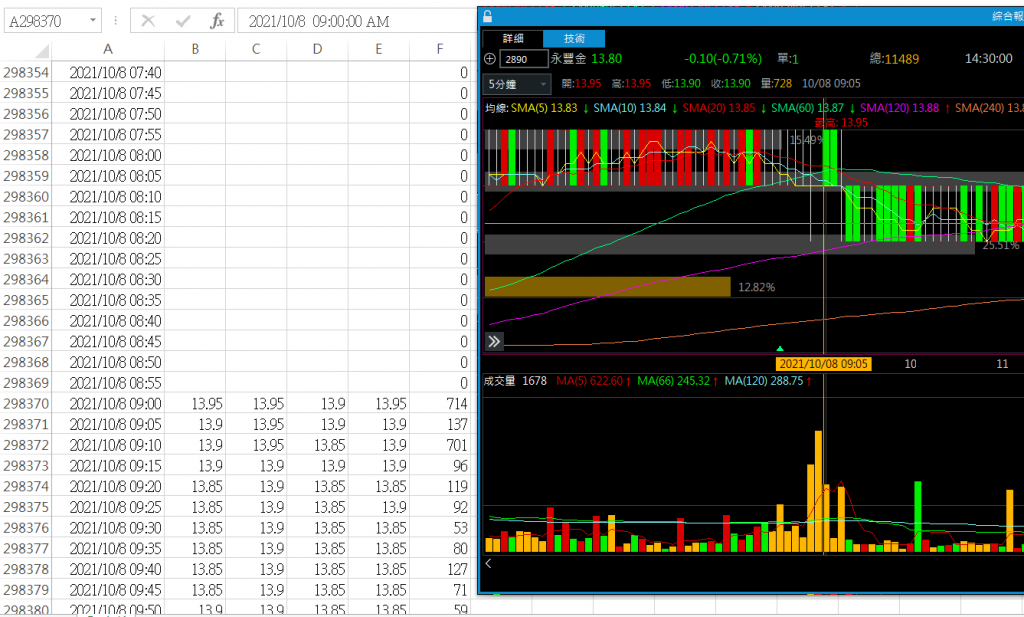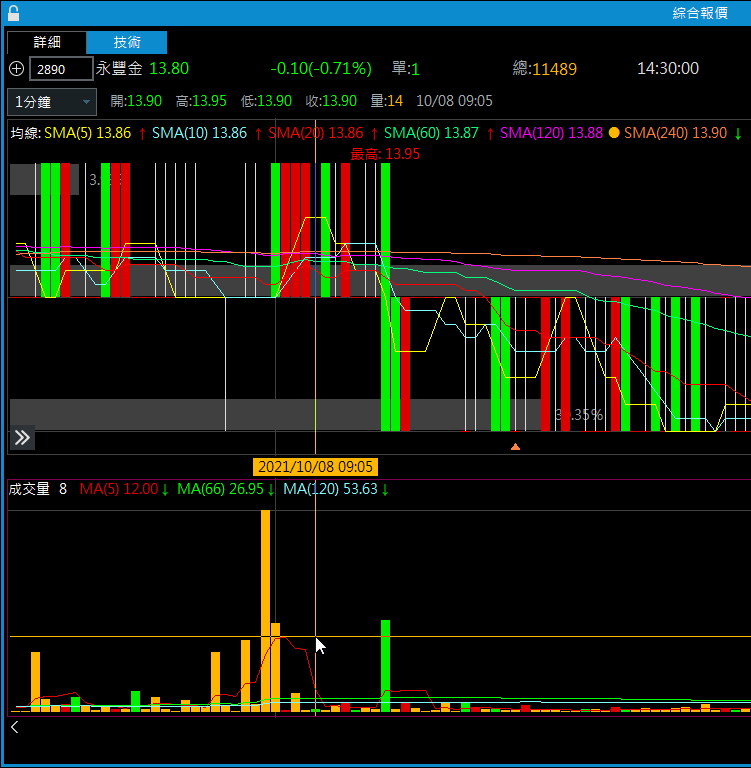``````# 執行resample，並指定close為right
df_5minK = df.resample(rule='5min', closed='right').agg({'Open': 'first',
'High': 'max',
'Low': 'min',
'Close': 'last',
'Volume': 'sum'})
``````

## DataFrame.resample中的Left與Right

`關於DataFrame.resample中的Left與Right，在官方的說明文件中並沒有特別說明，以下為我個人的理解`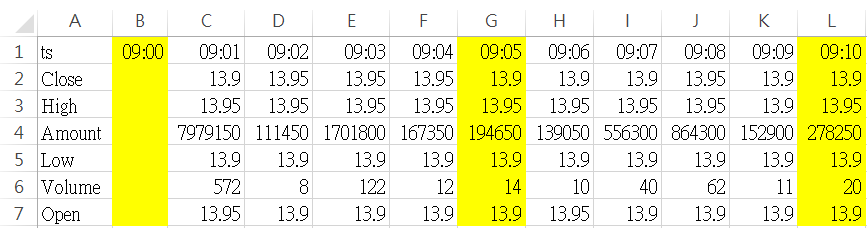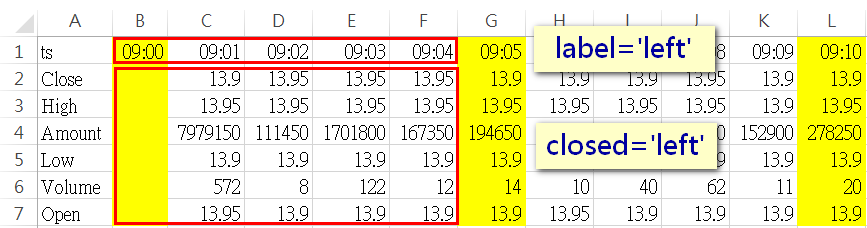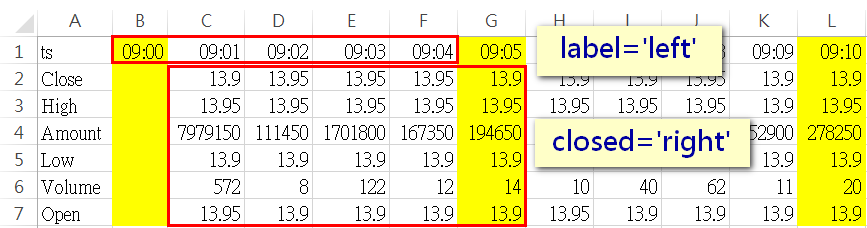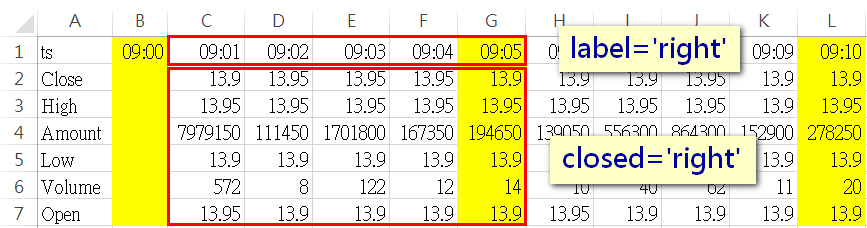## 使用DataFrame.dropna移除資料

pandas.DataFrame.dropna來刪除這些不需要的資料

``````df_5minK.dropna(axis=0, inplace=True) #移除有空值的row，並取代原本的DataFrame
``````

## 產生5分K資料並儲存至資料庫

``````import pandas as pd
import sqlite3

conn = sqlite3.connect('D:/shioaji.db') #建立資料庫連線

# 傳入股票代碼，產生5分K資料並存至資料庫
def generate_5min_kbar(stock_code):
print(f'generate 5minK for stock:{stock_code}') #輸出程式執行點資料至console
df = pd.read_sql(f'SELECT code, Open, High, Low, Close, Volume, ts FROM stocks_1min_kbars WHERE code = {stock_code}', conn, parse_dates=['ts'])
df.set_index('ts', inplace=True)
df_5min_kbar = df.resample(rule='5min', closed='right').agg({
'code': 'first',
'Open': 'first',
'High': 'max',
'Low': 'min',
'Close': 'last',
'Volume': 'sum'})
df_5min_kbar.dropna(axis=0, inplace=True)
df_5min_kbar.to_sql('stocks_5min_kbars', conn, if_exists='append')
print(f'stock:{stock_code}, 5minK is added to DB...') #輸出程式執行點資料至console

cursor = conn.cursor() #建立cursor物件
# 執行SQL，取出stocks_1min_kbars中所有的股票代碼(不重複)
for code in cursor.execute('SELECT DISTINCT code FROM stocks_1min_kbars'):
generate_5min_kbar(code)

conn.close() #關閉資料庫連線
``````